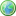Accessibility options:

# Completing the square resources

Show me all resources applicable to

### Test Yourself (6)Completing the square - Numbas
Two questions on completing the square. The first asks you to express $x^2+ax+b$ in the form $(x+c)^2+d$ for suitable numbers $c$ and $d$. The second asks you to complete the square on the quadratic of the form $ax^2+bx+c$ and then find its roots. Numbas resources have been made available under a Creative Commons licence by the School of Mathematics & Statistics at Newcastle University.Completing the square - Numbas
Two questions on completing the square. The first asks you to express $x^2+ax+b$ in the form $(x+c)^2+d$ for suitable numbers $c$ and $d$. The second asks you to complete the square on the quadratic of the form $ax^2+bx+c$ and then find its roots. Numbas resources have been made available under a Creative Commons licence by Bill Foster and Christian Perfect, School of Mathematics & Statistics at Newcastle University.Diagnostic Test - Completing the square 1
Diagnostic test for completing the square. (1 of 2). This resource is released under a Creative Commons license Attribution-Non-Commercial-No Derivative Works and the copyright is held by Skillbank Solutions Ltd.Diagnostic Test - Completing the square 2
Diagnostic test for completing the square. (2 of 2). This resource is released under a Creative Commons license Attribution-Non-Commercial-No Derivative Works and the copyright is held by Skillbank Solutions Ltd.Exercise - Completing the square
Online exercise on completing the square. This resource is released under a Creative Commons license Attribution-Non-Commercial-No Derivative Works and the copyright is held by Skillbank Solutions Ltd.Maths EG
Computer-aided assessment of maths, stats and numeracy from GCSE to undergraduate level 2. These resources have been made available under a Creative Common licence by Martin Greenhow and Abdulrahman Kamavi, Brunel University.

Website design by Pink Mayhem, Leicester